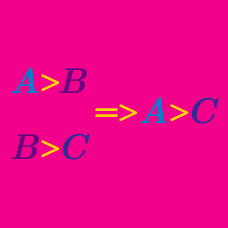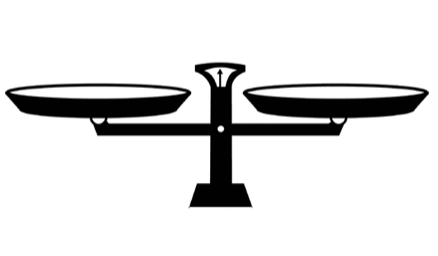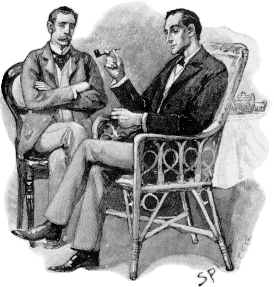Logic

# Logical Reasoning: Level 3 ChallengesYou are given 9 coins which look identical, but one is lighter than the rest. Using the balance scale above, what is the minimum number of weighings you need to guarantee that you can find the counterfeit?You are on a train with 4 other people, one of whom stole your wallet!

Here are their statements, and exactly 3 of them are lies:

• Person 1 said, "2 stole the wallet."
• Person 2 said, "3 didn't steal it."
• Person 3 said, "I didn't steal it."
• Person 4 said, "3 stole your wallet."There is a total of 9 eggs, out of which 8 have identical weights and one has a different weight. We do not know if the different egg is lighter or heavier.

Using a balance, what is the minimum number of weighings required to guarantee that you'll be able to determine the egg with different weight?

Suppose you have 8 watermelons that are exactly the same in appearance. They are equal in weight except for one, which is heavier than the others. You want to find the heaviest one by using a two-pan balance. Each pan can hold any number of watermelons, and the only items that you can place in the pans are watermelons.

Find the best strategy to determine the heaviest watermelon. In the worst case scenario, how many times do you have to use the two-pan balance?

1. Exactly one statement on this list is false.
2. Exactly two statements on this list are false.
3. Exactly three statements on this list are false.
4. Exactly four statements on this list are false.
5. Exactly five statements on this list are false.
6. Exactly six statements on this list are false.
7. Exactly seven statements on this list are false.
8. Exactly eight statements on this list are false.
9. Exactly nine statements on this list are false.
10. Exactly ten statements on this list are false.

How many statements from the above list are false?

×